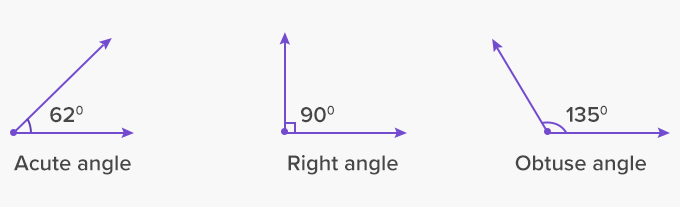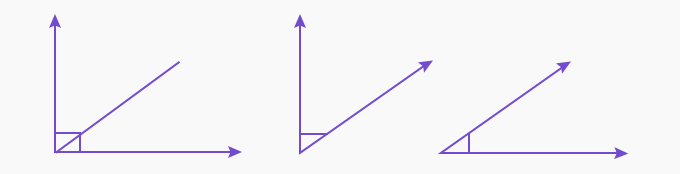# Acute Angle - Definition with Examples

The Complete K-5 Math Learning Program Built for Your Child

• 40 Million Kids

Loved by kids and parent worldwide

• 50,000 Schools

Trusted by teachers across schools

• Comprehensive Curriculum

Aligned to Common Core

## What is Acute Angle?

In geometry, two rays sharing a common endpoint form an angle. The common endpoint is called the vertex, and the two rays are called the arms of the angle.## Types of Angles

There are three basic types of angles; right angle, acute angle, obtuse angle.## Definition of an acute angle:

An acute angle is an angle that measures less than 90 degrees.∠ABC measures 30 ̊and hence it is an acute angle

A triangle formed by all angles measuring less than 90˚ is also known as an acute triangle. For example, in an equilateral triangle, all three angles measure 60˚, making it an acute triangle.Acute triangle

Dividing the right angle will give us two or more acute angles since each newly formed angle will be less than 90˚.

• Right Angle

• Two acute angles formed by dividing the right angleSome real-life examples of acute angles are:

If we slice a pizza into 5 or more slices, each slice of pizza will make an acute angle.Each slice of the pizza makes an acute angle.

Another example is the wall clock. The arms of a wall clock make acute angles at several hours of a day.  For example, 2 o’ Clock.The hour hand and the minute hand forming an acute angle at 2 o’ Clock.

 Fun Facts At least two angles of any triangle are acute angles. In a 180˚angle, if one angle is obtuse (more than 90˚), the other will always be an acute angle (less than 90˚).

Won Numerous Awards & Honors